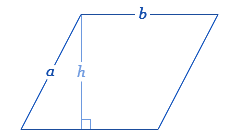# Perimeter of a Parallelogram Formula

The perimeter is the sum of the length of all the 4 sides. The perimeter of a parallelogram is the total distance outside the geometrical shape. The opposite sides of a parallelogram are equal and so the perimeter becomes twice the sum of two parallel sides say a and b.The perimeter of a Parallelogram Formula is given as,

Perimeter of a Parallelogram Formula = 2 (a + b) units

### Solved Example

Question:

Find the perimeter of a parallelogram whose base is 8 cm and another side is 12 cm.

Solution:

Given,

b = 8 cm

a = 12 cm

Perimeter of a Parallelogram = 2(a + b)

= 2(12 + 8)

= 2 x 20 cm

= 40 cm